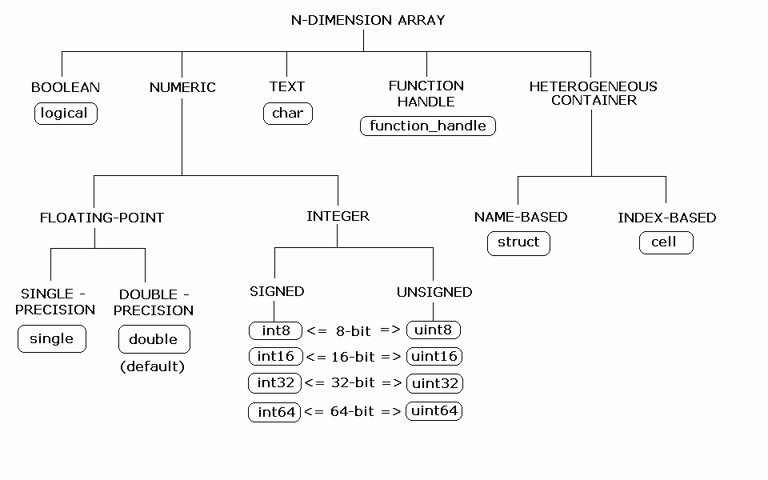Documentation

### This is machine translation

Mouseover text to see original. Click the button below to return to the English version of the page.

To view all translated materials including this page, select Country from the country navigator on the bottom of this page.

## Data Conversion with C# and MATLAB Types

When the .NET client invokes a MATLAB® function through a request and receives a result in the response, data conversion takes place between MATLAB types and C# types.

### Working with MATLAB Data Types

There are many data types, or classes, that you can work with in MATLAB. Each of these classes is in the form of a matrix or array. You can build matrices and arrays of floating-point and integer data, characters and strings, and logical true and false states. Structures and cell arrays provide a way to store dissimilar types of data in the same array.

All of the fundamental MATLAB classes are circled in the diagram Fundamental MATLAB Data Classes.

### Note

Function Handles are not supported by MATLAB Production Server™.

Fundamental MATLAB Data ClassesEach MATLAB data type has a specific equivalent in C#. Detailed descriptions of these one-to-one relationships are defined in Conversion Between MATLAB Types and C# Types.

### Scalar Numeric Type Coercion

Scalar numeric MATLAB types can be assigned to multiple .NET numeric types as long as there is no loss of data or precision.

The main exception to this rule is that MATLAB `double` scalar data can be mapped into any .NET numeric type. Because `double` is the default numeric type in MATLAB, this exception provides more flexibility to the users of MATLAB Production Server .NET client API.

MATLAB to .NET Numeric Type Compatibility describes the type compatibility for scalar numeric coercion.

MATLAB to .NET Numeric Type Compatibility

MATLAB Type.NET Types
`uint8``System.Int16`, `System.UInt16`, `System.Int32`, `System.UInt32`, `System.Int64`, `System.UInt64`, `System.Single`, `System.Double`
`int8``System.Int16`, `System.Int32`, `System.Int64`, `System.Single`, `System.Double`
`uint16``System.Int32`, `System.UInt32`, `System.Int64`, `System.UInt64`, `System.Single`, `System.Double`
`int16``System.Int32`, `System.Int64`, `System.Single`, `System.Double`
`uint32``System.Int64`, `System.UInt64`, `System.Single`, `System.Double`
`int32``System.Int64`, `System.Single`, `System.Double`
`uint64``System.Single`, `System.Double`
`int64``System.Single`, `System.Double`
`single``System.Double`
`double``System.SByte`, `System.Byte`, `System.Int16`, `System.UInt16`, `System.Int32`, `System.UInt32`, `System.Int64`, `System.UInt64`, `System.Single`

### Dimension Coercion

In MATLAB, dimensionality is an attribute of the fundamental types and does not add to the number of types as it does in .NET.

In C#, `double`, `double[]` and `double[,]` are three different data types. In MATLAB, there is only a `double` data type and possibly a scalar instance, a vector instance, or a multi-dimensional instance.

C# SignatureValue Returned from MATLAB
`double[,,] foo() ``ones(1,2,3)`

How you define your MATLAB function and corresponding C# method signature determines if your output data will be coerced, using padding or truncation.

This coercion is performed automatically for you. This section describes the rules followed for padding and truncation.

### Note

Multidimensional arrays of C# types are supported. Jagged arrays are not supported.

When a C# method's return type has a greater number of dimensions than MATLAB’s, MATLAB's dimensions are padded with ones (`1`s) to match the required number of output dimensions in C#.

The following tables provide examples of how padding is performed for you:

How Your C# Method Return Type is Padded

MATLAB FunctionC# Method SignatureWhen Dimensions in MATLAB are:And Dimensions in C# are:
`function a = fooa = ones(2,3);``double[,,,] foo() ``size(a)` is `[2,3]`Array will be returned as size `2,3,1,1`

#### Truncation

When a C# method's return type has fewer dimensions than MATLAB’s, MATLAB’s dimensions are truncated to match the required number of output dimensions in C#. This is only possible when extra dimensions for MATLAB array have values of ones (`1`s) only.

To compute appropriate number of dimensions in C#, excess ones are truncated, in this order:

1. From the end of the array

2. From the array’s beginning

3. From the middle of the array (scanning front-to-back).

The following tables provide examples of how truncation is performed for you:

How MATLAB Truncates Your C# Method Return Type

MATLAB FunctionC# Method SignatureWhen Dimensions in MATLAB are:And Dimensions in C# are:
`function a = fooa = ones(1,2,1,1,3,1);``double[,] foo()``size(a)` is `[1,2,1,1,3,1]`Array will be returned as size `2,3`

Following are some examples of dimension shortening using the ```double numeric``` type:

Truncating Dimensions in MATLAB and C# Data Conversion

MATLAB Array DimensionsDeclared Output C# TypeOutput C# Dimensions
`1 x 1``double`0 (scalar)
`2 x 1``double[]``2`
`1 x 2``double[]``2`
`2 x 3 x 1``double[,]``2 x 3`
`1 x 3 x 4``double[,]``3 x 4`
`1 x 3 x 4 x 1 x 1``double[,,]``1 x 3 x 4`
`1 x 3 x 1 x 1 x 2 x 1 x 4 x 1``double[,,,]``3 x 2 x 1 x 4`

### Empty (Zero) Dimensions

##### SupportGet trial now